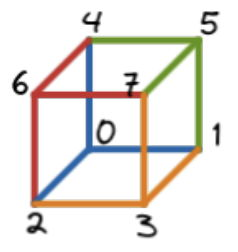시간 제한메모리 제한제출정답맞힌 사람정답 비율
1 초 512 MB0000.000%

## 문제

...it’s dark in the cube, it’s dark in the cube...

Five in the morning. Daniel wakes up, he opens his eyes. His head hurts a bit. He can still hear the ringing in his ears.

He comes to realize that he has found himself at a playground, in a big metal box.

...I was in the cube, I was in the cube...

He remembers a similar situation he found himself in, three years ago, COCI round 2, task Kocka.

...I’m in the cube again, I’m in the cube again...

But this time, things are much more complicated... Daniel is in an $n$-dimensional hipercube $\mathcal{Q}_n$. $2^{n-1}$ identical copies of a tree $\mathcal{T}$ with $n$ edges are scattered around him. It soon became clear to him that salvation lies in tiling the edges of the hipercube with the trees.

Formally, a hipercube $\mathcal{Q}_n$ is a graph with nodes $0$, $1$, $\dots$, $2^n-1$, in which nodes $x$ and $y$ are connected if and only if their bitwise xor is a power of two.

A tree can be placed on the hipercube so that:

• each node of the tree corresponds to some node of the hipercube
• those nodes have to be distinct
• if there is an edge between two nodes in the tree, then there has to be an edge between the corresponding nodes in the hipercube.

A tiling of the hipercube is done by placing several trees so that each edge of the hipercube belongs to at most one tree.

Your task is to tile the hipercube $\mathcal{Q}_n$ with as many copies of the given tree $\mathcal{T}$, which has $n$ edges.

## 입력

The first line contains a positive integer $n$ ($1 ≤ n ≤ 16$), the dimension of the hipercube.

Each of the following $n$ lines contains two integers $x$ and $y$ ($0 ≤ x, y ≤ n$, $x \ne y$) which denote that the nodes $x$ and $y$ are connected by an edge in tree $\mathcal{T}$.

## 출력

In the first line print the number of trees in your tiling.

Each of the following lines should describe a placement of a single copy of the tree $\mathcal{T}$.

In the $i$-th line print $n + 1$ numbers $a_0^{(i)}$, $a_1^{(i)}$, $\ldots$ $a_n^{(i)}$. These numbers denote that the $i$-th tree is placed so that the hipercube node $a_j^{(i)}$ corresponds to the tree node $j$, for all $j = 0, \dots , n$.

## 점수

If your solution correctly places $k$ trees, you will receive $f(k) \cdot 110$ points for that test case, where

$f(k) = \begin{cases}0.7 \cdot k / 2^{n-1} & \text{if } k < 2^{n-1} \\ 1 & \text{if } k = 2^{n-1}\end{cases}$.

Of course, if your solution is not correct, you will receive $0$ points.

Your total number of points is equal to the minimum number of points your solution receives over all of the test cases.

It is possible to prove that there always exists a solution which uses all of the $2^{n-1}$ trees.

## 예제 입력 1

1
0 1


## 예제 출력 1

1
0 1


## 예제 입력 2

2
0 1
1 2


## 예제 출력 2

2
0 1 3
0 2 3


## 예제 입력 3

3
0 1
0 2
0 3


## 예제 출력 3

4
0 1 2 4
3 1 2 7
5 1 4 7
6 2 4 7


## 힌트

Clarification of the third example:## 채점 및 기타 정보

• 110점 이상을 획득해야 를 받는다.